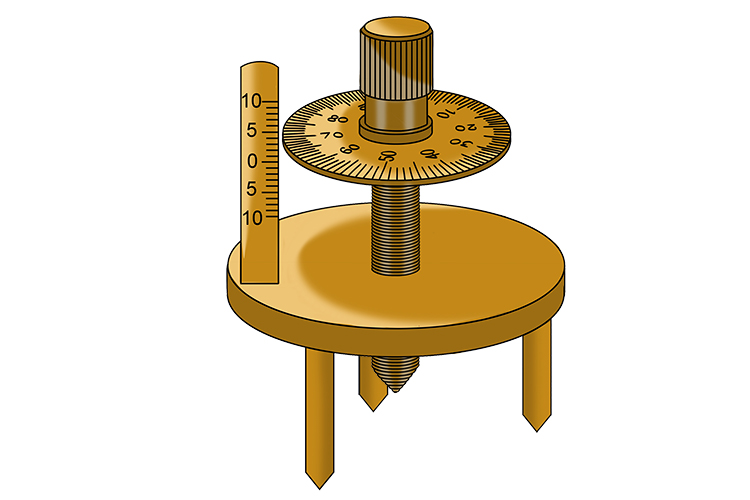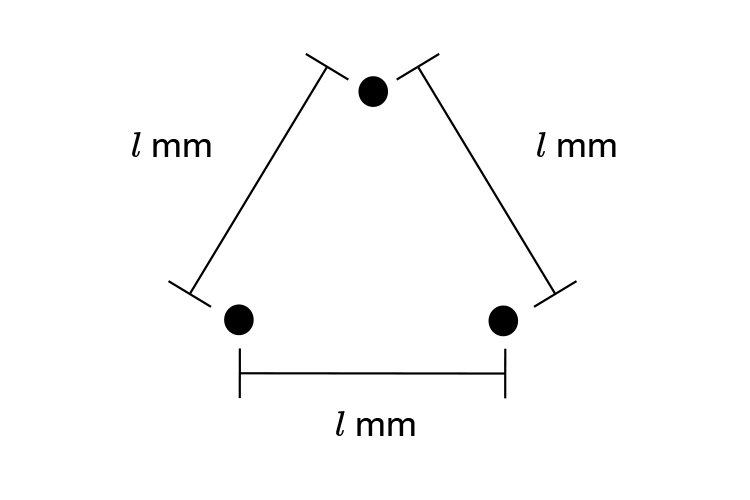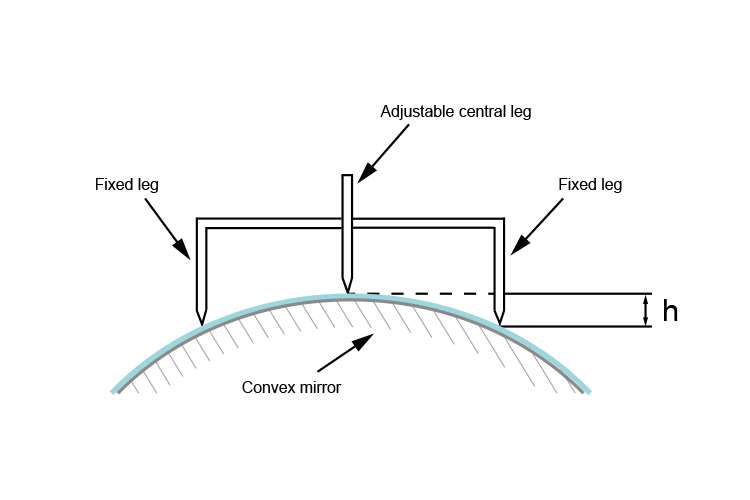# Practical way to find the principal focal length – Spherometer

You will never need the information below for physics at this level. Read on only for interest's sake.

A spherometer is a device which does exactly as it says: a sphere (sphere) it measures (ometer). It is a device used to measure the curvature of a sphere. See below:The spherometer has three straight legs equally spaced out, like the corner points of an equilateral triangle. A fourth leg is at the centre of the triangle and is adjustable.

The surface of the convex mirror is round like the back of a spoon and so the central leg must be shorter and must be adjusted up and down until all four tips touch the surface at the same time.

A circular disk with a scale marked around its outer edge is fixed to the head of the screw. You can read the height difference between the fixed legs and the adjustable leg. One turn is equal to 1mm.

The height difference is directly related to the radius of curvature.

A formula is used to convert this height difference to the radius of curvature of the sphere.lmm is the distance between each of the three fixed legs.hmm is the reading on the spherometer and is the height.

Again we emphasise that you do not need this formula to pass basic physics exams but here is the formula you would use:

The radius of curvature =l^2/(6h)+h/2

and also remember that:

The principal focal length =R/2